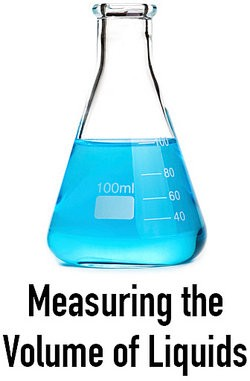# Examples of Ways to Measure Volume

Volume is the level at which something is heard or the amount of space a solid, liquid or gas occupies.

• With sound, its volume is the loudness of the sound.

• With a container, its volume would be its capacity, or how much it can hold.

Volume is often expressed in cubic units determined by the International System of Units.

## Measuring the Volume of Sound

The loudness of a sound can be subjective, such as quiet or loud. A more objective measure of sound includes several other factors including:

• Sound pressure level (SPL) - The human ear averages the SPL over a period of 600-1000 milliseconds. After about 1 second, the ear created an average and the level of loudness will seem to become stable.

• Decibels (dB) - This is the ratio between the power and intensity of the sound. The maximum human sensitivities are between 2 and 4 kHz.

## Measuring the Volume of Solids

The volume of solids is expressed in cubic measurements, such as cubic centimeter or cubic meter.

Here are examples of ways to measure solids:

• Rectangular prism - Multiply the measurement of the length times the width, then times the height

• Cube - Since all sides are the same measurement, it would be the measurement of any side, or edge, cubed, or a³

• Prism - Find the area of the base, then multiply the area times the height of the prism. Area would be length times width (B), or B x h

• Triangular prism - Find the area of the base (b), then multiply the area times the length (l) between the triangular bases. Area of the base would be ½ times the base times the height (h) of the triangle, or ½bhl

• Cylinder - Find the area of the circular face using pi times the radius squared, then multiply that area times the height of the cylinder, so πr²h

• Pyramid - Find the area of the base and multiply that area times the height of the pyramid and multiply by 1/3, or 1/3Bh

• Square pyramid - Find the area of the base and multiply that area times the height of the pyramid and multiply by 1/3, or 1/3s²h

• Rectangular pyramid - Find the area of the base and multiply that area times the height of the pyramid and multiply by 1/3, or 1/3 lwh

• Sphere - For a sphere, multiply 4/3 times pi, then time the radius cubed, or 4/3 πr³

• Cone - For a cone, it is the area of the circular face, or πr², then multiply by 1/3 and the height of the cone, or 1/3 πr²h

## Measuring the Volume of Liquids

Liquids are fairly simple to measure, remembering that 1000 cubic centimetres equals 1 litre and 1000 litres equals 1 cubic metre.

Here are examples of ways to measure liquids:

• Barrels - Barrels can be dry barrels or fluid barrels, such as oil and water barrels. The specific amount of volume a barrel holds has differed over time and depending on the type.

In Europe, the term "barrel" as a unit of measure has ranged from signifying around 100 litres to signifying around 1,000 litres.

In the US, a beer barrel is around 31 gallons while an oil barrel is around 42 gallons.

• Gallons - A gallon is equal to 128 ounces; four quarts; eight pints and around 3.781 liters.

• Measuring cups - Measuring cups come in various sizes and can be used to measure liquid or dry goods. A set of measuring cups for dry goods usually includes a ¼ of a cup, a ⅓ of a cup, a ½ cup and a 1-cup measure.

• Measuring spoons - Measuring spoons also come in various sizes and can measure dry or liquid goods. A standard set of measuring spoons generally contains a ¼ teaspoon measure; a ½ teaspoon measure; a 1 teaspoon measure; and a 1 tablespoon measure.

• Pints - A pint is equal to 1 milliliter, .125 gallons, and .5 quarts.

• Quarts- A quart is equal to 946.353 milliliters, .25 gallons and four quarts.

## Measuring the Volume of Gases

Here are examples of ways to measure volume of gases:

• Displace fluid - This method can be used to calculate the volume of gas. The volume of fluid displaced would equal the volume of the gas.

• Use a gas syringe - This is a laboratory instrument that can be used to withdraw a certain volume of gas from a closed system or to measure the volume of gas from a chemical reaction.

• Use a spirometer - It measures the volume of air coming into and being expelled out of your lungs, called tidal volume.

• Trap the gas - Use a balloon to trap the gas coming from a reaction. Then calculate the volume of the sphere.

• For the volume of compressed gas in a cylinder, apply this equation, the Ideal Gas Law: PV = nRT, where P is pressure, V is volume, n is the number of moles, R is the gas constant and T is the absolute temperature

Now you have learned plenty of different ways to measure volume.Examples of Ways to Measure Volume